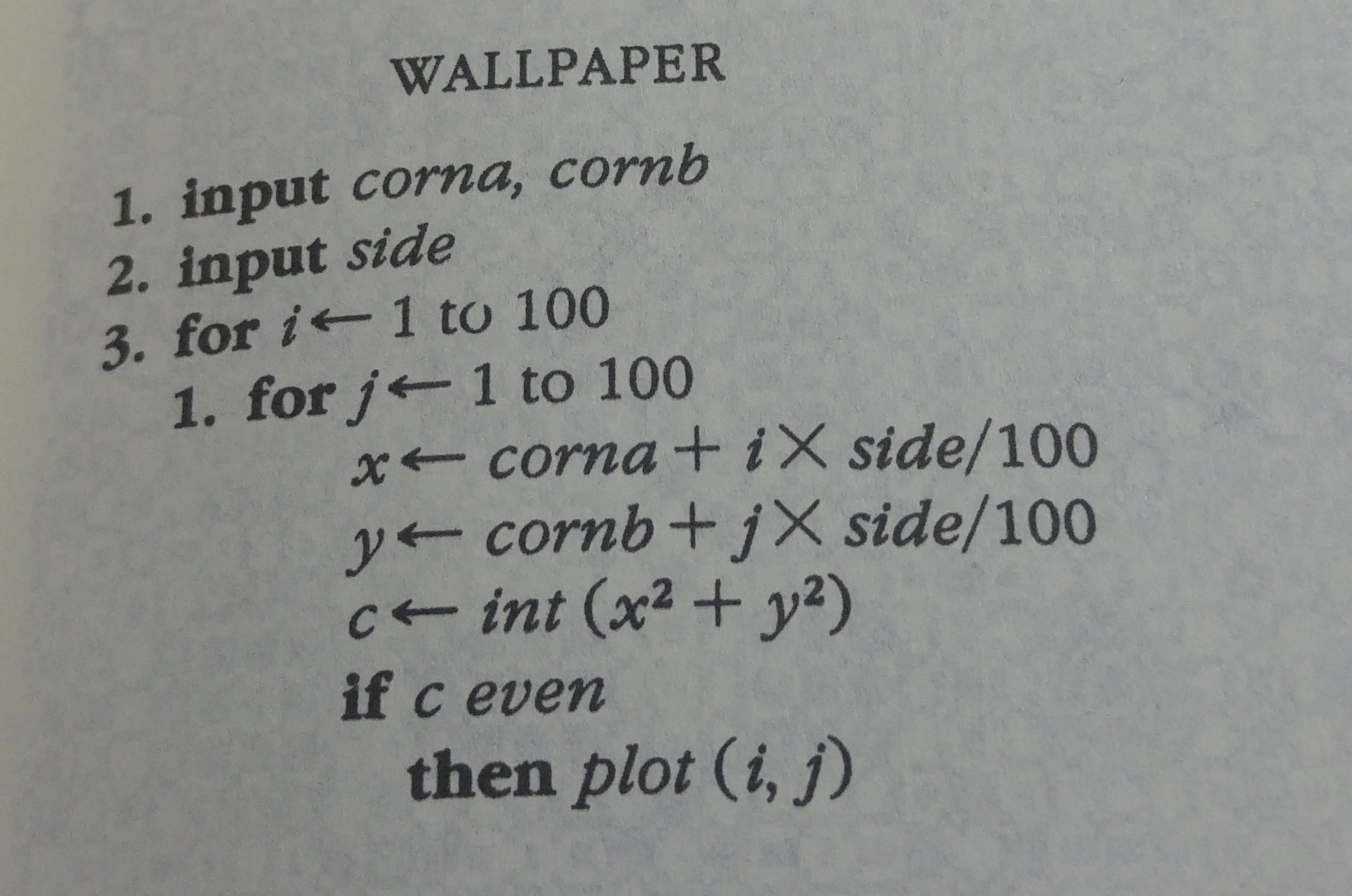I have started to read The New Turing Omnibus - a book that offers 66 concise, brilliantly written articles on the major points of interest in computer science theory, technology and applications.

From time to time, I will write a blog post presenting a chapter of this book.Today, I am glad to present an interactive version of Chapter 1 about algorithms in general.

## The algorithm

In order to explain what is an algorithm, the author presents a simple recipe for generating wallpapers.

Here is the recipe:Now, we are going to code this algorithm in `javascript` and you will be able to play with it right in your browser thanks to the interactive Klipse snippets.

## Preliminaries

First, we need a function that draws a color pixel on a canvas:

``````function drawPixel(canvas, x, y, color) {
let ctx = canvas.getContext("2d"),
scale = 2;
ctx.fillStyle = color;
ctx.fillRect(x * scale, y * scale, scale, scale)
}
``````

Then, a function that erases a canvas i.e. color it in white:

``````function resetCanvas (canvas){
let ctx = canvas.getContext("2d");
ctx.fillStyle = "white";
ctx.fillRect(0, 0, canvas.width,canvas.height);
};
``````

## Black and White Wallpaper

The algorithm is controlled by the geometry of a square:

• its `x`-position named `a`
• its `y`-position named `b`
• the side of the square named `side`
``````

function drawBwWallpaper(canvas, a, b, side){
const points = 200;
for(let i=0; i< points; i++)
for(let j=0; j< points; j++) {
const x = a + i * side/points;
const y = b + j * side/points;
const c = Math.floor(x*x + y*y);
if (c % 2 === 0) {
drawPixel(canvas, i, j, "black");
}
}
}

drawBwWallpaper(canvas, 5, 5, 9);
``````

The cool thing about this algorithm is that when we modify the side of the square, we get a completly different pattern:

``````

drawBwWallpaper(canvas, 5, 5, 100)
``````

Go ahead, play with the code…

The interactive code snippets are powered by the Klipse plugin.

## Three Colors

We can generate a 3-color wallpaper by calculating the remainder of `c` modulo 4 and chose a color accordingly:

``````
function choseColor(c) {
let colors = ["red", "green", "blue", "white"];
return colors[c % 4];
}
function drawColorWallpaper(canvas, a, b, side){
const points = 200;
for(let i=0; i<points; i++){
for(let j=0; j<points; j++) {
const x = a + i * side/points;
const y = b + j * side/points;
const c = Math.floor(x*x + y*y);
drawPixel(canvas, i, j, choseColor(c));
}
}
}
drawColorWallpaper(canvas, 5, 7, 100)
``````

Again, when we modify the side of the square, we get a completly different pattern:

``````
drawColorWallpaper(canvas, 5, 7, 57)
``````

## Grand Finale

Someone in reddit suggested to loop over the value of `side` in order to watch all the generated wallpapers like a movie.

Here is the result:

``````
if(! window.interval) {
window.interval = null;
}
let side = 0;
const delta = 0.5;
function step(canvas, container) {
container.innerHTML = `side: \${side}`;
resetCanvas(canvas);
drawColorWallpaper(canvas, 5, 7, side);
side += delta;
}

clearInterval(window.interval);
window.interval = setInterval(step, 500, canvas, klipse_container);
``````

Are you able to provide a simple explanation about this algorithm?

How is it able to generate so many different beautiful patterns?

Have you found a magnificient pattern? Please share its code…